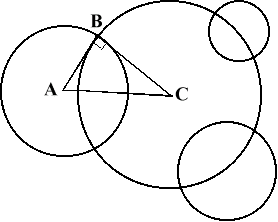#### You may also like### Baby Circle

A small circle fits between two touching circles so that all three circles touch each other and have a common tangent? What is the exact radius of the smallest circle?### Kissing

Two perpendicular lines are tangential to two identical circles that touch. What is the largest circle that can be placed in between the two lines and the two circles and how would you construct it?### Logosquares

Ten squares form regular rings either with adjacent or opposite vertices touching. Calculate the inner and outer radii of the rings that surround the squares.

# Orthogonal Circle

##### Age 16 to 18Challenge LevelIt is known that given any three non intersecting circles in the plane there is another circle or straight line that cuts the three given circles at right angles. (The circle or straight line is said to be orthogonal to the 3 original circles.) Given three circles with centres $(0, 0)$, $(3, 0)$ and $(9, 2)$ and radii $5$, $4$ and $6$ respectively find the centre and radius of the circle that cuts the three given circles at right angles. Draw the circles to check that the circle you have found appears to be orthogonal to the others.

What happens in the case of three circles with centres at $(0, 0)$, $(3, 3)$ and $(8, 8)$ and radii $1$, $2$ and $3$ respectively?

Given three circles, how can you tell without calculating which of the two cases applies, an orthogonal circle or an orthogonal straight line?